# 30.5: Biosynthesis

$$\newcommand{\vecs}{\overset { \rightharpoonup} {\mathbf{#1}} }$$ $$\newcommand{\vecd}{\overset{-\!-\!\rightharpoonup}{\vphantom{a}\smash {#1}}}$$$$\newcommand{\id}{\mathrm{id}}$$ $$\newcommand{\Span}{\mathrm{span}}$$ $$\newcommand{\kernel}{\mathrm{null}\,}$$ $$\newcommand{\range}{\mathrm{range}\,}$$ $$\newcommand{\RealPart}{\mathrm{Re}}$$ $$\newcommand{\ImaginaryPart}{\mathrm{Im}}$$ $$\newcommand{\Argument}{\mathrm{Arg}}$$ $$\newcommand{\norm}{\| #1 \|}$$ $$\newcommand{\inner}{\langle #1, #2 \rangle}$$ $$\newcommand{\Span}{\mathrm{span}}$$ $$\newcommand{\id}{\mathrm{id}}$$ $$\newcommand{\Span}{\mathrm{span}}$$ $$\newcommand{\kernel}{\mathrm{null}\,}$$ $$\newcommand{\range}{\mathrm{range}\,}$$ $$\newcommand{\RealPart}{\mathrm{Re}}$$ $$\newcommand{\ImaginaryPart}{\mathrm{Im}}$$ $$\newcommand{\Argument}{\mathrm{Arg}}$$ $$\newcommand{\norm}{\| #1 \|}$$ $$\newcommand{\inner}{\langle #1, #2 \rangle}$$ $$\newcommand{\Span}{\mathrm{span}}$$$$\newcommand{\AA}{\unicode[.8,0]{x212B}}$$

## Fatty Acids

The idea that ethanoic acid (acetic acid) is a possible common starting material for the biosynthesis of many organic compounds was first proposed by Collie (1893) on purely structural grounds. He recognized a structural connection between a linear chain of recurring $$\ce{CH_3CO}$$ units (a polyketomethylene chain, $$\ce{CH_3COCH_2COCH_2COCH_2CO}-$$) and certain cyclic natural products. In the example given below, orsellinic acid is represented as if it were derived from a chain of four $$\ce{CH_3CO}$$ units by a condensation-cyclization reaction: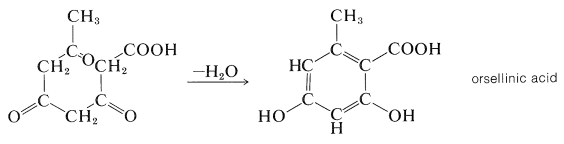Experimental verification of Collie's hypothesis came many years later when isotopic hydrogen and carbon ($$\ce{^2H}$$, $$\ce{^3H}$$, $$\ce{^{13}C}$$, and $$\ce{^{14}C}$$) became available. Tracer studies showed that long-chain fatty acids are made by plants and animals from $$\ce{CH_3CO}$$ units by successively linking together the carbonyl group of one to the methyl group of another (K. Bloch and F. Lynen, Nobel Prize, 1964). If ethanoic acid supplied to the organism is labeled at the carboxyl group with $$\ce{^{14}C} \: \left( \overset{*}{\ce{C}} \right)$$, the fatty acid has the label at alternate carbons:

$\ce{CH_3} \overset{*}{\ce{C}} \ce{H_2CH_2} \overset{*}{\ce{C}} \ce{H_2CH_2} \overset{*}{\ce{C}} \ce{H_2CH_2} \overset{*}{\ce{C}} \ce{H_2CH_2} \overset{*}{\ce{C}} \ce{H_2CH_2} \overset{*}{\ce{C}} \ce{H_2CH_2} \overset{*}{\ce{C}} \ce{O_2H}$

However, if the carbon of the methyl group is labeled, the product comes out labeled at the other set of alternate carbons:

$\overset{*}{\ce{C}} \ce{H_3CH_2} \overset{*}{\ce{C}} \ce{H_2CH_2} \overset{*}{\ce{C}} \ce{H_2CH_2} \overset{*}{\ce{C}} \ce{H_2CH_2} \overset{*}{\ce{C}} \ce{H_2CH_2} \overset{*}{\ce{C}} \ce{H_2CH_2} \overset{*}{\ce{C}} \ce{H_2CO_2H}$

Ethanoic acid is activated for biosynthesis by combination with the thiol, coenzyme A (\textbf{CoA} \ce{SH}\), Figure 18-7) to give the thioester, ethanoyl (acetyl) coenzyme A $$\left( \ce{CH_3COS} \textbf{CoA} \right)$$. You may recall that the metabolic degradation of fats also involves this coenzyme (Section 18-8F) and it is tempting to assume that fatty acid biosynthesis is simply the reverse of fatty acid metabolism to $$\ce{CH_3COS} \textbf{CoA}$$. However, this is not quite the case. In fact, it is a general observation in biochemistry that primary metabolites are synthesized by different routes from those by which they are metabolized (for example, compare the pathways of carbon in photosynthesis and metabolism of carbohydrates in Sectionss 20-9 and 20-10).

A brief description of the main events in fatty-acid biosynthesis follows, and all of these steps must be understood to be under control of appropriate enzymes and their coenzymes even though they are omitted here.

The $$\ce{CH_3CO}$$ group of ethanoyl coenzyme A is first transferred to a protein having a free thiol $$\left( \ce{SH} \right)$$ group to make another thioester, represented here as $$\ce{CH_3COS}-\textbf{ACP}$$, where ACP stands for Acyl-Carrier-Protein. The growing carbon chain remains bound to this protein throughout the synthesis:

$\ce{CH_3COS} \textbf{CoA} + \ce{HS}-\textbf{ACP} \rightarrow \ce{CH_3COS}-\textbf{ACP} + \textbf{CoA} \ce{SH}$

Carboxylation of $$\ce{CH_3COS}-\textbf{ACP}$$ yields a propanedioyl thioester, $$6$$, which then undergoes a Claisen condensation with a second mole of $$\ce{CH_3COS}-\textbf{ACP}$$ accompanied by decarboxylation to yield a 3-oxobutanoyl thioester, $$7$$:Reduction of the ketone group of the thioester (by $$\ce{NADPH}$$) leads to a thiol ester of a four-carbon carboxylic acid. Repetitive condensations with thioester $$6$$ followed by reduction eventually lead to fatty acids. Each repetition increases the chain length by two carbons:The preceding scheme is representative of fatty acid biosynthesis in plants, animals, and bacteria. The major difference is that plant and bacterial fatty acids usually contain more double bonds (or even triple bonds) than do animal fatty acids.

## Biosynthesis of Aromatic Rings

Collie's hypothesis that aromatic compounds are made biologically from ethanoic acid was greatly expanded by A. J. Birch to include an extraordinary number of diverse compounds. The generic name "acetogenin" has been suggested as a convenient classification for ethanoate (acetate)-derived natural products, but the name "polyketides" also is used. Naturally occurring aromatic compounds and quinones are largely made in this way. An example is 2-hydroxy-6-methylbenzoic acid formed as a metabolite of the mold Penicillium urticae; using $$\ce{^{14}C}$$-carboxyl-labeled ethanoic acid, the label has been shown to be at the positions indicated below:## Terpene Biosynthesis

The biosynthesis of terpenes clearly follows a somewhat different course from fatty acids in that branched-chain compounds are formed. One way that this can come about is for 2-oxobutanoyl coenzyme A to undergo an aldol addition at the keto carbonyl group with the ethanoyl coenzyme A to give the 3-methyl-3-hydroxypentanedioic acid derivative, $$8$$:The next step is reduction of one of the carboxyl groups of $$8$$ to give mevalonic acid: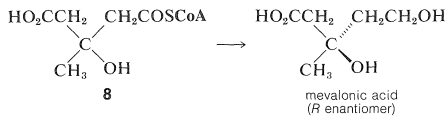This substance has been shown by tracer studies to be an efficient precursor of terpenes and steroids. Mevalonic acid has six carbon atoms, whereas the isoprene unit has only five. Therefore, if mevalonic acid is the precursor of isoprene units, it must lose one carbon atom at some stage. Synthesis of mevalonic acid labeled at the carboxyl group with $$\ce{^{14}C}$$, and use of this material as a starting material for production of cholesterol, gives unlabeled cholesterol. Therefore, the carboxyl carbon is the one that is lost: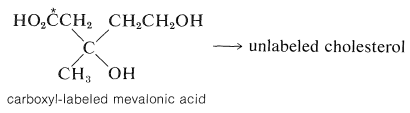Formation of the "biological isoprene unit" from mevalonic acid has been shown to proceed by stepwise phosphorylation of both alcohol groups, then elimination and decarboxylation to yield 3-methyl-3-butenyl pyrophosphate, $$9$$ (often called $$\Delta^3$$-isopentenyl pyrophosphate):The coupling of the five-carbon units, $$9$$, to give isoprenoid compounds has been suggested to proceed by the following steps. First, isomerization of the double bond is effected by an enzyme $$\left( \ce{E} \right)$$ carrying an $$\ce{SH}$$ group: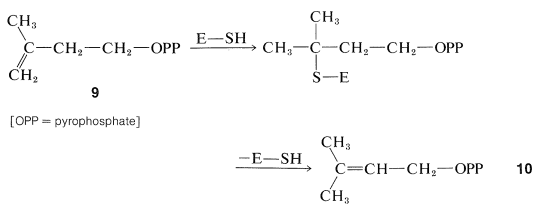The ester, $$10$$, then becomes connected to the double bond of a molecule of $$9$$, probably in an enzyme-induced carbocation type of polymerization (Section 10-8B):The product of the combination of two units of the pyrophosphate, $$9$$, through this sequence is geranyl pyrophosphate if, as shown, the proton is lost to give a trans double bond. Formation of a cis double bond would give neryl pyrophosphate (Section 30-3B).

Continuation of the head-to-tail addition of five-carbon units to geranyl (or neryl) pyrophosphate can proceed in the same way to farnesyl pyrophosphate and so to gutta-percha (or natural rubber). At some stage, a new process must be involved because, although many isoprenoid compounds are head-to-tail type polymers of isoprene, others, such as squalene, lycopene, and $$\beta$$- and $$\gamma$$-carotene (Table 30-1), are formed differently. Squalene, for example, has a structure formed from head-to-head reductive coupling of two farnesyl pyrophosphates:Since squalene can be produced from farnesyl pyrophosphate with $$\ce{NADPH}$$ and a suitable enzyme system, the general features of the above scheme for terpene biosynthesis are well supported by experiment.

In summary, the sequence from ethanoate to squalene has been traced as

$\text{ethanoyl coenzyme A} \rightarrow \text{mevalonic acid} \rightarrow \text{isopentenyl pyrophosphate} \rightarrow \text{farnesyl pyrophosphate} \rightarrow \text{squalene}$

## Cholesterol Biosynthesis

Isotopic labeling experiments show that cholesterol is derived from ethanoate by way of squalene and lanosterol. The evidence for this is that homogenized liver tissue is able to convert labeled squalene to labeled lanosterol and thence to labeled cholesterol. The conversion of squalene to lanosterol is particularly interesting because, although squalene is divisible into isoprene units, lanosterol is not - a methyl being required at $$\ce{C_8}$$ and not $$\ce{C_{13}}$$:As a result, some kind of rearrangement must be required to get from squalene to lanosterol. The nature of this rearrangement becomes clearer if we write the squalene formula so as to take the shape of lanosterol:When squalene is written in this form, we see that it is beautifully constructed for cyclization to lanosterol. The key intermediate that initiates the cyclization is the 2,3-epoxide of squalene. Enzymatic cleavage of the epoxide ring is followed by cyclization and then manifold hydride $$\left( \ce{H} \colon \right)$$ and methide $$\left( \ce{CH_3} \colon \right)$$ shifts to give lanosterol: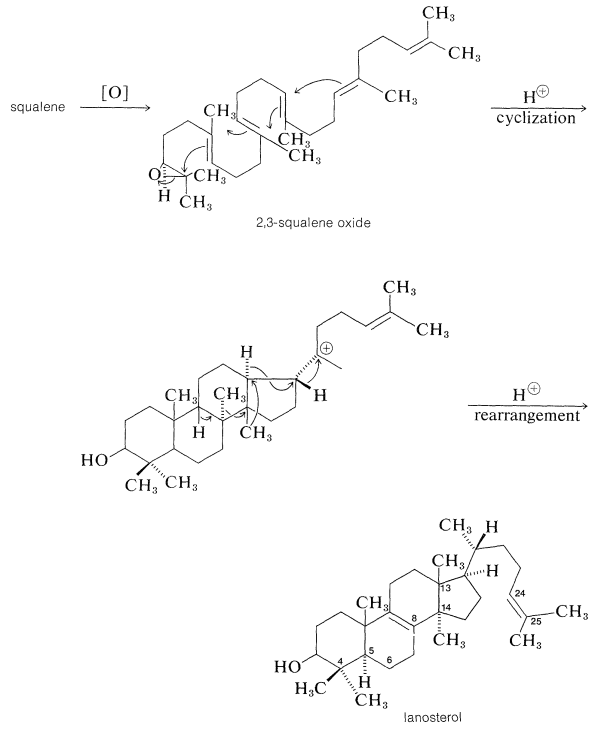The evidence is strong that the biosynthesis of lanosterol actually proceeds by a route of this type. With squalene made from either methyl- or carboxyl-labeled ethanoate, all the carbons of lanosterol and cholesterol are labeled just as predicted from the mechanism. Furthermore, ingenious double-labeling experiments have shown that the methyl at $$\ce{C_{13}}$$ of lanosterol is the one that was originally located at $$\ce{C_{14}}$$, whereas the one at $$\ce{C_{14}}$$ is the one that came from $$\ce{C_8}$$.

The conversion of lanosterol to cholesterol involves removal of the three methyl groups at the 4,4- and 14-positions, shift of the double bond at the $$B$$/$$C$$ junction between $$\ce{C_5}$$ and $$\ce{C_6}$$, and reduction of the $$\ce{C_{24}}$$-$$\ce{C_{25}}$$ double bond. The methyl groups are indicated by tracer experiments to be eliminated by oxidation to carbon dioxide.

The biosynthetic connection between ethanoyl coenzyme A and the complex natural products briefly discussed is summarized in Figure 30-1.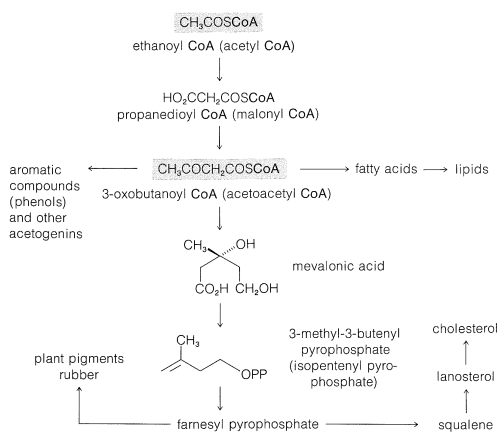Figure 30-1: Summary of biosynthesis pathways to fatty acids, terpenes, and steroids.